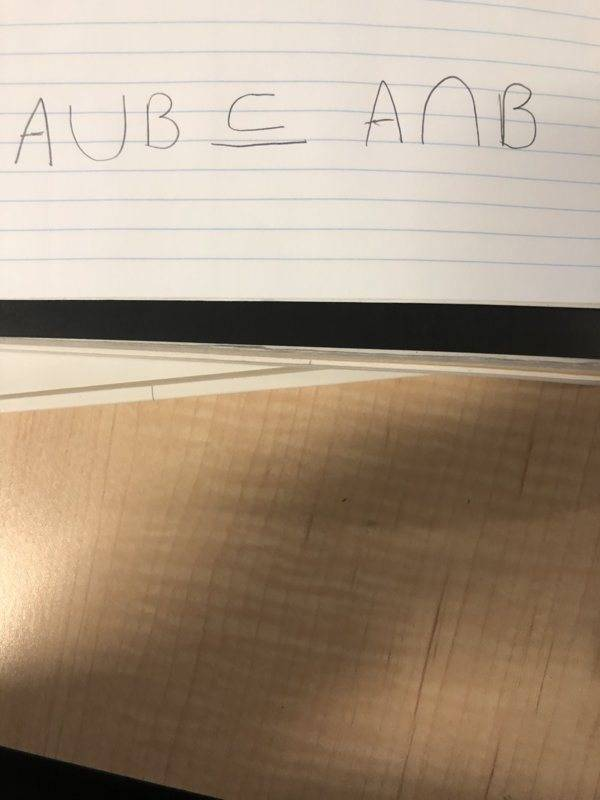# This would be a false statement, correct?

• I
EchoRush
TL;DR Summary
A quick question about sets and intersection/unions
I believe that I am correct, the following statement here must be FALSE, right? It has to be false because A union B is like the two entire circles of the Venn diagram and that cannot be a subset of the intersection area, right? Now if this statement was flipped, then it would be true?Homework Helper
Correct!

Homework Helper
By the way PF would really appreciate typed posts instead of disk-space wasting big pictures !

If you type
##A\cup B \subseteq A\cap B## then you get ##A\cup B \subseteq A\cap B## (in-line math), and if you type
$$A\cup B \subseteq A\cap B$$ then you get $$A\cup B \subseteq A\cap B$$
('displayed math').

•Mark44 and Dale
Yes correct. For example take ##A = \{0\}, B = \{1\}##. Then ##A\cup B = \{0,1\}## yet ##A \cap B = \emptyset##.

The other inclusion, that is ##A \cap B \subseteq A \cup B##, is trivially true.

Homework Helper
Gold Member
2022 Award
Summary:: A quick question about sets and intersection/unions

I believe that I am correct, the following statement here must be FALSE, right? It has to be false because A union B is like the two entire circles of the Venn diagram and that cannot be a subset of the intersection area, right? Now if this statement was flipped, then it would be true?

You can, however, have ##A \cup B = A \cap B##, so it's not always false. Can you see when you have this equality?

It is correct that ##A \cup B## can never be a proper subset of ##A \cap B##.

•FactChecker and pbuk
Homework Helper
Gold Member
You can, however, have ##A \cup B = A \cap B##, so it's not always false. Can you see when you have this equality?
Yes, absolutely, it is NOT correct to say that the statement is unconditionally false.

I believe that I am correct, the following statement here must be FALSE, right?
To reinforce what others have said, there is a difference between talking about a "statement" versus taking about a "propositional function".

If you utter a phrase like "If x > 7 then x > 1" , it is technically a "propositional function", which cannot be assigned a truth value ( True vs False) until "x" is defined to be something specific. In common speech and in informal mathematical writing, it is usually understood that phrases like "If x >7 then x > 1" are intended to have the "universal quantifier" given by the phrase "for each". So the reader interprets the claim "If x > 7 then x > 1" to mean "For each number x, if x >7 then x > 1". With this interpretation, the phrase becomes a statement which can be assigned a truth value.

The other commonly used quantifier is "there exists". Someone writing hasty notes might jot down the phrase "x > 1" intending it to mean "There exists a number x such that x > 1". However, this is not a clear style of writing.

Your question about "##A \cup B \subseteq A \cap B##" is technically a question about a propositional function, so it cannot be assigned a single truth value. You probably intended some universal quantifiers to be supplied, so the phrase would become the statement "For each set ##A## and for each set ##B##, ## A \cup B \subseteq A \cap B##". With the universal quantifiers, the propositional function is converted into a False statement. However, if we supply existential quantifiers, we get "There exists a set ##A## and there exists a set ##B## such that ## A \cup B \subseteq A \cap B##". This converts the propositional function into a True statement.

••PeroK and pbuk